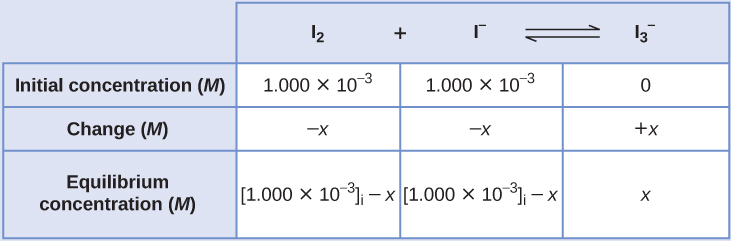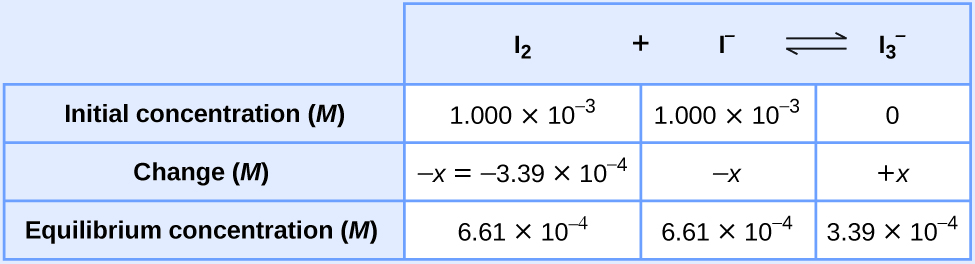# 13.4 Equilibrium calculations  (Page 3/11)

 Page 3 / 11

## Calculation of an equilibrium constant

Iodine molecules react reversibly with iodide ions to produce triiodide ions.

${\text{I}}_{2}\left(aq\right)+{\text{I}}^{\text{−}}\left(aq\right)⇌{\text{I}}_{3}{}^{\text{−}}\left(aq\right)$

If a solution with the concentrations of I 2 and I both equal to 1.000 $×$ 10 −3 M before reaction gives an equilibrium concentration of I 2 of 6.61 $×$ 10 −4 M , what is the equilibrium constant for the reaction?

## Solution

We will begin this problem by calculating the changes in concentration as the system goes to equilibrium. Then we determine the equilibrium concentrations and, finally, the equilibrium constant. First, we set up a table with the initial concentrations, the changes in concentrations, and the equilibrium concentrations using − x as the change in concentration of I 2 .Since the equilibrium concentration of I 2 is given, we can solve for x . At equilibrium the concentration of I 2 is 6.61 $×$ 10 −4 M so that

$1.000\phantom{\rule{0.2em}{0ex}}×\phantom{\rule{0.2em}{0ex}}{10}^{-3}-x=6.61\phantom{\rule{0.2em}{0ex}}×\phantom{\rule{0.2em}{0ex}}{10}^{-4}$
$x=1.000\phantom{\rule{0.2em}{0ex}}×\phantom{\rule{0.2em}{0ex}}{10}^{-3}-6.61\phantom{\rule{0.2em}{0ex}}×\phantom{\rule{0.2em}{0ex}}{10}^{-4}$
$=3.39\phantom{\rule{0.2em}{0ex}}×\phantom{\rule{0.2em}{0ex}}{10}^{-4}\phantom{\rule{0.2em}{0ex}}M$

Now we can fill in the table with the concentrations at equilibrium.We now calculate the value of the equilibrium constant.

${K}_{c}={Q}_{c}=\phantom{\rule{0.2em}{0ex}}\frac{\left[{\text{I}}_{3}{}^{\text{−}}\right]}{\left[{\text{I}}_{2}\right]\left[{\text{I}}^{\text{−}}\right]}$
$=\phantom{\rule{0.2em}{0ex}}\frac{3.39\phantom{\rule{0.2em}{0ex}}×\phantom{\rule{0.2em}{0ex}}{10}^{-4}\phantom{\rule{0.2em}{0ex}}M}{\left(6.61\phantom{\rule{0.2em}{0ex}}×\phantom{\rule{0.2em}{0ex}}{10}^{-4}\phantom{\rule{0.2em}{0ex}}M\right)\left(6.61\phantom{\rule{0.2em}{0ex}}×\phantom{\rule{0.2em}{0ex}}{10}^{-4}\phantom{\rule{0.2em}{0ex}}M\right)}\phantom{\rule{0.2em}{0ex}}=776$

Ethanol and acetic acid react and form water and ethyl acetate, the solvent responsible for the odor of some nail polish removers.

${\text{C}}_{2}{\text{H}}_{5}\text{OH}+{\text{CH}}_{3}{\text{CO}}_{2}\text{H}⇌{\text{CH}}_{3}{\text{CO}}_{2}{\text{C}}_{2}{\text{H}}_{5}+{\text{H}}_{2}\text{O}$

When 1 mol each of C 2 H 5 OH and CH 3 CO 2 H are allowed to react in 1 L of the solvent dioxane, equilibrium is established when $\frac{1}{3}$ mol of each of the reactants remains. Calculate the equilibrium constant for the reaction. (Note: Water is not a solvent in this reaction.)

K c = 4

## Calculation of a missing equilibrium concentration

If we know the equilibrium constant for a reaction and know the concentrations at equilibrium of all reactants and products except one, we can calculate the missing concentration.

## Calculation of a missing equilibrium concentration

Nitrogen oxides are air pollutants produced by the reaction of nitrogen and oxygen at high temperatures. At 2000 °C, the value of the equilibrium constant for the reaction, ${\text{N}}_{2}\left(g\right)+{\text{O}}_{2}\left(g\right)⇌2\text{NO}\left(g\right),$ is 4.1 $×$ 10 −4 . Find the concentration of NO( g ) in an equilibrium mixture with air at 1 atm pressure at this temperature. In air, [N 2 ] = 0.036 mol/L and [O 2 ] 0.0089 mol/L.

## Solution

We are given all of the equilibrium concentrations except that of NO. Thus, we can solve for the missing equilibrium concentration by rearranging the equation for the equilibrium constant.

${K}_{c}={Q}_{c}=\phantom{\rule{0.2em}{0ex}}\frac{{\left[\text{NO}\right]}^{2}}{\left[{\text{N}}_{2}\right]\phantom{\rule{0.2em}{0ex}}\left[{\text{O}}_{2}\right]}$
${\left[\text{NO}\right]}^{2}={K}_{c}\left[{\text{N}}_{2}\right]\phantom{\rule{0.2em}{0ex}}\left[{\text{O}}_{2}\right]$
$\left[\text{NO}\right]=\phantom{\rule{0.2em}{0ex}}\sqrt{{K}_{c}\left[{\text{N}}_{2}\right]\phantom{\rule{0.2em}{0ex}}\left[{\text{O}}_{2}\right]}$
$=\phantom{\rule{0.2em}{0ex}}\sqrt{\left(4.1\phantom{\rule{0.2em}{0ex}}×\phantom{\rule{0.2em}{0ex}}{10}^{-4}\right)\left(0.036\right)\left(0.0089\right)}$
$=\phantom{\rule{0.2em}{0ex}}\sqrt{1.31\phantom{\rule{0.2em}{0ex}}×\phantom{\rule{0.2em}{0ex}}{10}^{-7}}$
$=3.6\phantom{\rule{0.2em}{0ex}}×\phantom{\rule{0.2em}{0ex}}{10}^{-4}$

Thus [NO] is 3.6 $×$ 10 −4 mol/L at equilibrium under these conditions.

We can check our answer by substituting all equilibrium concentrations into the expression for the reaction quotient to see whether it is equal to the equilibrium constant.

${Q}_{c}=\phantom{\rule{0.2em}{0ex}}\frac{{\left[\text{NO}\right]}^{2}}{\left[{\text{N}}_{2}\right]\phantom{\rule{0.2em}{0ex}}\left[{\text{O}}_{2}\right]}$
$=\phantom{\rule{0.2em}{0ex}}\frac{{\left(3.6\phantom{\rule{0.2em}{0ex}}×\phantom{\rule{0.2em}{0ex}}{10}^{-4}\right)}^{2}}{\left(0.036\right)\left(0.0089\right)}$
${Q}_{c}=4.0\phantom{\rule{0.2em}{0ex}}×\phantom{\rule{0.2em}{0ex}}{10}^{-4}={K}_{c}$

The answer checks; our calculated value gives the equilibrium constant within the error associated with the significant figures in the problem.

The equilibrium constant for the reaction of nitrogen and hydrogen to produce ammonia at a certain temperature is 6.00 $×$ 10 −2 . Calculate the equilibrium concentration of ammonia if the equilibrium concentrations of nitrogen and hydrogen are 4.26 M and 2.09 M , respectively.

1.53 mol/L

how can someone understand chemistry vividly
Maybe by reading proofs or practical work and application in modern world.
Abdelkarim
what is isotopes
whats de shape of water
water has no shape because it's liquid
Wil
water is a shapeless, odourless, colourless and tasteless substance that only takes the shape of its container.
mikefred
i think they're referring to the molecular shape?
It has no shape but takes the shape of the container
what isthe maening pkw
introduction to chemistry for beginner
hi
noble
through out human history
Yes?
Esther
hello
noble
yes
Bol
what
noble
is euglena a unicellular organ
is euglena a unicellular organism
Agio
how is hydrogen can be heated
what is difference between atom and molecule
Atom is the smallest part of matter; it consists of equal number of protons and electrons. It may have neutrons. A molecule is a compound made of atoms covalently bonded.
Abdelkarim
does amoeba has structure
what is the effect of green house on the earth 🌎
what is the effect of green house on the earth 🌎
kolawole
what's an atom?
it's the smallest unit of Matter
Orsine
atom
Eden
smallest part of an element
lydia
also, depending on its (atom's) structure, that is the amount of protons and neutrons and electrons, is the determining factors of what element it is.
Richard
is a smallast particals of an element
Buwembo
it is the smallest part of an element that can take part in a chemical reaction
Ayub
is the smallest part of an element
Jonathan
An atom is the smallest indivisible part of a matter
Oy kl konsa test hay or kitna hay?
differences between solid liquid and gaseous state
modification of John dalton atomic theory
Ochei
the differences between soliq liquid and gas is that in solid the particle are strongly bonded together by forces of cohesion and the particle are not able to move about but only vibrate in a fixed position but in liquid the particle are loosely bond together and the particle are able to move about
kolawole
2.4g of magnesium reacts with 0.3mol of hydrochloric acid write a balanced chemical equation for the reaction. (b)Determine the limiting reactant

#### Get Jobilize Job Search Mobile App in your pocket Now!ByByBy Janet ForresterBy JavaChamp TeamBy Stephanie RedfernBy OpenStaxBy OpenStaxBy OpenStaxBy Anh DaoBy OpenStaxBy Brooke DelaneyBy Anonymous User